# Voltage Measurements: How-to Guide - What is Voltage?

## Overview

Learn the fundamentals of creating an AC or DC voltage measurement system. See how to properly connect the signals to your data acquisition system for accurate acquisition.

## Voltage Measurement OverviewVoltage is the difference of electrical potential between two points of an electrical or electronic circuit, expressed in volts.It measures the potential energy of an electric field to cause an electric current in an electrical conductor.

Most measurement devices can measure voltage. Two common voltage measurements are direct current (DC) and alternating current (AC). Although voltage measurements are the simplest of the different types of analog measurements, they present unique challenges due to noise considerations.

## How to Make a DC Voltage Measurement

Although many sensors output DC voltages that you can measure with a multimeter or data acquisition device, the primary concern of this white paper is to examine general DC measurements that do not involve an intermediary sensor setup.

### Voltage Measurement Fundamentals

To understand how to measure voltages, it is essential to understand the background of how you take the measurement. Essentially, voltage is the electrical potential difference between two points of interest in an electrical circuit. However, a common point of confusion is how the measurement reference point is determined. The measurement reference point is the voltage level at which the measurement is referenced to.

### Reference Point Methods

There are essentially two methods to measure voltages: ground referenced and differential.

#### Ground Referenced Voltage Measurement

One method is to measure voltage with respect to a common, or a “ground” point. Oftentimes, these “grounds” are stable and unchanging and are most commonly around 0 V. Historically, the term ground originated from the usual application of ensuring the voltage potential is at 0 V by connecting the signal directly to the earth.Ground referenced input connections are particularly good for a channel that meets the following conditions:

• The input signal is high-level (greater than 1 V)
• The leads connecting the signal to the device are less than 10 ft (3 m)
• The input signal can share a common reference point with other signals

The ground reference is provided by either the device taking the measurement or by the external signal being measured. When the ground is provided by the device, this setup is called ground referenced single-ended mode (RSE), and when the ground is provided by the signal, the setup is called non-referenced single-ended mode (NRSE).

Most instruments offer similar pin configurations for analog input measurements. The following example demonstrates this type of measurement using an CompactDAQ chassis and an NI 9205 analog input module (see Figure 1).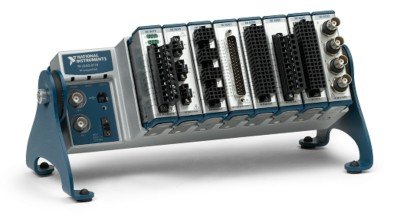Figure 1. CompactDAQ Chassis with an NI 9205 Analog Input Module

Figure 2 shows the connection diagram for RSE voltage measurements using an NI cDAQ-9178 chassis with an NI 9205 as well as the pinouts for the module. In Figure 2, Pin 1 corresponds to the “Analog Input 0” channel and Pin 17 corresponds to the common ground.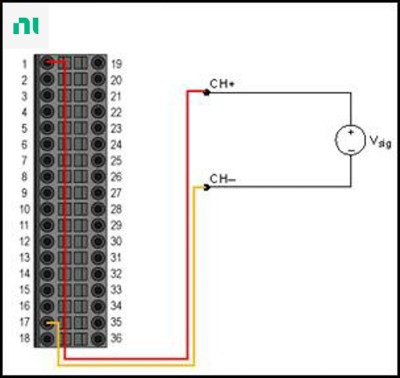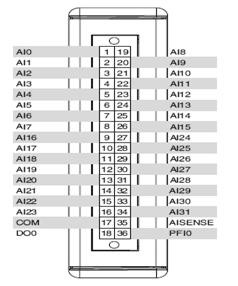Figure 2. Ground Referenced Single-Ended Mode

Figure 3 shows the connection diagram for NRSE voltage measurements using a cDAQ-9178 with an NI 9205. In the figure, Pin 1 corresponds to the “Analog Input 0” channel and Pin 35 corresponds to the “Analog Input Sense” channel. This channel, specifically for NRSE measurements, can detect the ground voltage provided by the signal.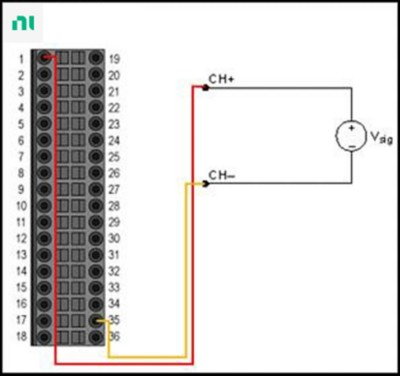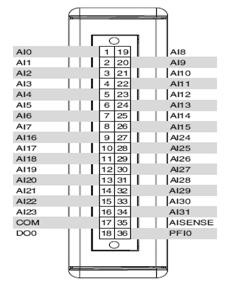Figure 3. Non-referenced Single-Ended Mode

#### Differential Voltage Measurement

Another way to measure voltage is to determine the “differential” voltage between two separate points in an electrical circuit. For example, to measure the voltage across a single resistor, you measure the voltage at both ends of the resistor. The difference between the voltages is the voltage across the resistor. Usually, differential voltage measurements are useful in determining the voltage that exists across individual elements of a circuit, or if the signal sources are noisy.

Differential input connections are particularly well-suited for a channel that meets any of the following conditions:

• The input signal is low-level (less than 1 V)
• The leads connecting the signal to the device are greater than 3 m (10 ft)
• The input signal requires a separate ground reference point or return signal
• The signal leads travel through noisy environments

Figure 4 illustrates the connection diagram for differential voltage measurements using a cDAQ- 9178 with an NI 9205. In the figure, Pin 1 corresponds to the “Analog Input 0” channel and Pin 19 corresponds to the “Analog Input 8” channel.

In differential mode, the negative signal is wired to the analog pin directly facing the analog channel that is connected to the positive signal. For example, “Analog Input 0” would be connected to positive, and “Analog Input 8” would be connected to the negative signals, and “Analog Input 1” for positive and “Analog Input 9” for negative and so on. The disadvantage of differential mode is that it effectively reduces the number of analog input measurement channels by half.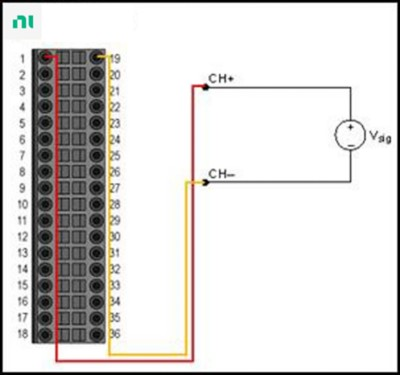.Figure 4. Differential Mode

### Types of Signal Sources

Before configuring the input channels and making signal connections, you must determine whether the signal sources are floating or ground referenced.

#### Floating Signal Sources

A floating signal source is not connected to the building ground system but has an isolated ground reference point. Some examples of floating signal sources are outputs of transformers, thermocouples, battery-powered devices, optical isolators, and isolation amplifiers. An instrument or device that has an isolated output is a floating signal source. The ground reference of a floating signal must be connected to the ground of the device to establish a local or onboard reference for the signal. Otherwise, the measured input signal varies as the source floats outside the common-mode input range.

#### Ground Referenced Signal Sources

A ground referenced signal source is connected to the building system ground, so it is already connected to a common ground point with respect to the device, assuming that the measurement device is plugged into the same power system as the source. Non-isolated outputs of instruments and devices that plug into the building power system fall into this category. The difference in ground potential between two instruments connected to the same building power system is typically between 1 and 100 mV, but the difference can be much higher if power distribution circuits are improperly connected. If a grounded signal source is incorrectly measured, this difference can appear as measurement error. Following the connection instructions for grounded signal sources can eliminate the ground potential difference from the measured signal.

Figure 5 shows the different types of signal source types as well as the optimal connection diagrams based on the individual measurement method. Please note that depending on the type of signal, a particular voltage measurement method may provide better results than others.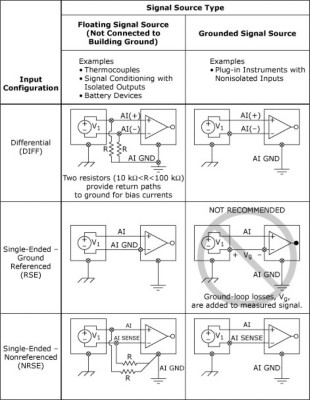Figure 5. Common Signal Source Types versus Recommended Input Configurations

Learn more about Field Wiring and Noise Considerations for Analog Signals.

### High-Voltage Measurements and Isolation

There are many issues to consider when measuring higher voltages. When specifying a data acquisition system, the first question you should ask is whether the system will be safe. Making high-voltage measurements can be hazardous to your equipment, to the unit under test, and even to you and your colleagues. To ensure that your system is safe, you should provide an insulation barrier between the user and hazardous voltages with isolated measurement devices.

Isolation, a means of physically and electrically separating two parts of a measurement device, can be categorized into electrical and safety isolation. Electrical isolation pertains to eliminating ground paths between two electrical systems. By providing electrical isolation, you can break ground loops, increase the common-mode range of the data acquisition system, and level shift the signal ground reference to a single system ground. Safety isolation references standards that have specific requirements for isolating humans from contact with hazardous voltages. It also characterizes the ability of an electrical system to prevent high-voltage and transient voltages to be transmitted across its boundary to other electrical systems with which the user may come in contact.

Incorporating isolation into a data acquisition system has three primary functions: preventing ground loops, rejecting common-mode voltage, and providing safety.

### Ground Loops

Ground loops are the most common source of noise in data acquisition applications. They occur when two connected terminals in a circuit are at different ground potentials, causing current to flow between the two points. The local ground of your system can be several volts above or below the ground of the nearest building, and nearby lightning strikes can cause the difference to rise to several hundreds or thousands of volts. This additional voltage itself can cause significant error in the measurement, but the current that causes it can couple voltages in nearby wires as well. These errors can appear as transients or periodic signals. For example, if a ground loop is formed with 60 Hz AC power lines, the unwanted AC signal appears as a periodic voltage error in the measurement.

When a ground loop exists, the measured voltage, Vm, is the sum of the signal voltage, Vs, and the potential difference,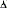Vg, which exists between the signal source ground and the measurement system ground (see Figure 6). This potential is generally not a DC level; thus, the result is a noisy measurement system often showing power-line frequency (60 Hz) components in the readings.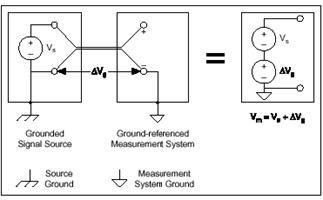Figure 6. A Grounded Signal Source Measured with a
Ground Referenced System Introduces Ground Loops

To avoid ground loops, ensure that there is only one ground reference in the measurement system, or use isolated measurement hardware. Using isolated hardware eliminates the path between the ground of the signal source and the measurement device, thus preventing any current from flowing between multiple ground points.

In the previously mentioned CompactDAQ setup, the NI 9229 analog input module provides 250 V channel-to-channel isolation.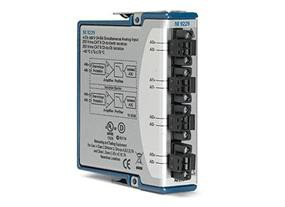Figure 7. NI 9229 Channel-to-Channel Isolated Analog Input Module

### Common-Mode Voltage

An ideal differential measurement system responds only to the potential difference between its two terminals, the (+) and (-) inputs. The differential voltage across the circuit pair is the desired signal, yet an unwanted signal may exist that is common to both sides of a differential circuit pair. This voltage is known as common-mode voltage. An ideal differential measurement system completely rejects, rather than measures, the common-mode voltage. Practical devices, however, have several limitations, described by parameters such as common-mode voltage range and common-mode rejection ratio (CMRR), which limit this ability to reject the common-mode voltage.

The common-mode voltage range is defined as the maximum allowable voltage swing on each input with respect to the measurement system ground. Violating this constraint results not only in measurement error but also in possible damage to components on the board.

Common-mode rejection ratio describes the ability of a measurement system to reject common-mode voltages. Amplifiers with higher common-mode rejection ratios are more effective at rejecting common-mode voltages.

In a non-isolated differential measurement system, an electrical path still exists in the circuit between input and output. Therefore, the electrical characteristics of the amplifier limit the common-mode signal level that you can apply to the input. With the use of isolation amplifiers, the conductive electrical path is eliminated and the common-mode rejection ratio is dramatically increased.

### Isolation Topologies

It is important to understand the isolation topology of a device when configuring a measurement system. Different topologies have several associated cost and speed considerations.

#### Channel-to-Channel

The most robust isolation topology is channel-to-channel isolation. In this topology, each channel is individually isolated from one another and from other non-isolated system components. In addition, each channel has its own isolated power supply.

In terms of speed, there are several architectures from which to choose. Using an isolation amplifier with an analog-to-digital converter (ADC) per channel is typically faster because you can access all of the channels in parallel. The NI 9229 and NI 9239 analog input modules provide channel-to-channel isolation to deliver the highest measurement accuracy.

A more cost-effective but slower architecture involves multiplexing each isolated input channel into a single ADC.

Another method of providing channel-to-channel isolation is to use a common isolated power supply for all of the channels. In this case, the common-mode range of the amplifiers is limited to the supply rails of that power supply, unless you use front-end attenuators.

#### Bank

Another isolation topology involves banking, or grouping, several channels together to share a single isolation amplifier. In this topology, the common-mode voltage difference between channels is limited, but the common-mode voltage between the bank of channels and the non-isolated part of the measurement system can be large. Individual channels are not isolated, but banks of channels are isolated from other banks and from ground. This topology is a lower-cost isolation solution because this design shares a single isolation amplifier and power supply.

Most NI C Series analog input modules, such as the NI 9201 and NI 9221, are bank-isolated and can provide accurate analog voltage measurements at a lower cost.

### Getting to See Your Measurement: NI LabVIEW

Once you have connected the sensor to the measurement instrument, you can use LabVIEW graphical programming software to visualize and analyze data as needed. See Figure 8, which is taken from the shipped LabVIEW Example: Voltage - Finite Input.vi and found here:

\National Instruments\<LabVIEW Version>\examples\DAQmx\Analog Input\Voltage - Finite Input.vi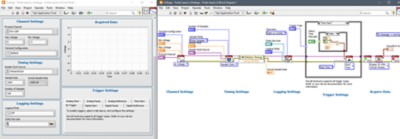Figure 8. LabVIEW Voltage Measurement